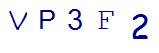# Three 6th Grade Geometry Activities with Real Life ExamplesLast Updated on May 26, 2022 by Thinkster

“Why do we need this?” This is a common question asked by 6th grade geometry students. If you want to get your sixth grader excited about math class, consider these examples that use real-world problems to teach geometry concepts.

## 1. Baseball Field Measurement

Baseball fields are full of shapes that can be measured and areas that can be computed, as well as angles to be determined. All of these work well in 6th grade geometry. Find a diagram that shows the layout of a baseball diamond complete with standard measurements, then use that to figure the perimeter of the bases, the angle of the home base to an outfield position and other figures you can determine. The more creative you are, the more opportunities to explore geometry on the baseball field you will find. Then, take your work outside to a real baseball field and see if the measurements add up.

## 2. Finding the Volume of a Box

Volume is a valuable tool when you are trying to fill a box. You can show your child this with a simple problem. Choose a small box and have an item to fill it with that is of uniform shapes, such as small boxes of crackers.

Ask your child to figure out how many boxes the larger box will hold without counting them. This will require first finding the volume of the small boxes by multiplying the length times width times height, then figuring out the volume of the large box, and finally determining how many of the smaller boxes will fit within that volume.

## 3. Pizza Deals

Geometry can actually help you save money when buying a pizza. Consider if you are buying a square 6-inch personal pizza or a slice of pizza from the grocery store. If the slice of pizza was cut from an 18-inch pizza, and the 18-inch pizza was cut into eight equal pieces, which is a better deal if both cost \$2?

In order to figure out a better deal, you need to figure out which serving is more pizza. The 6-inch square pizza is 36 square inches of pizza. To figure out how much pizza the slice is, you must first figure out the area of the full circular pizza. The formula for area is pi times the radius squared. The radius of the 18-inch pizza is 9, giving you an area of approximately 254 square inches. Divide this by eight for each slice, and you have a total amount of pizza of 31 inches. The square pizza is more pizza and the better deal.

Each of these 6th grade geometry examples uses real-world applications that kids will face in daily life. Using problems like this will get your kids excited about math by showing the “why” behind the processes they are learning. If you want to get your child even more excited about 6th grade geometry, consider investing in Thinkster Math, a digital learning program that instills excitement while promoting learning in children. With Thinkster, your child can get ahead in math while enjoying the learning process.

SummaryArticle Name
3 6th Grade Geometry Activities with Real Life Examples
Description
Concepts taught in 6th grade geometry often seem irrelevant to children. Here are 3 examples of showing these concepts at work in the real-world.
Author
Publisher Name
Thinkster Math
Publisher Logo

## Subscribe to Thinkster Blog## Recommended Articles### 6 Educational Math Games to Supplement Online Math Tutoring

Online math tutoring can help your student progress in their math learning - no matter their age, current l...